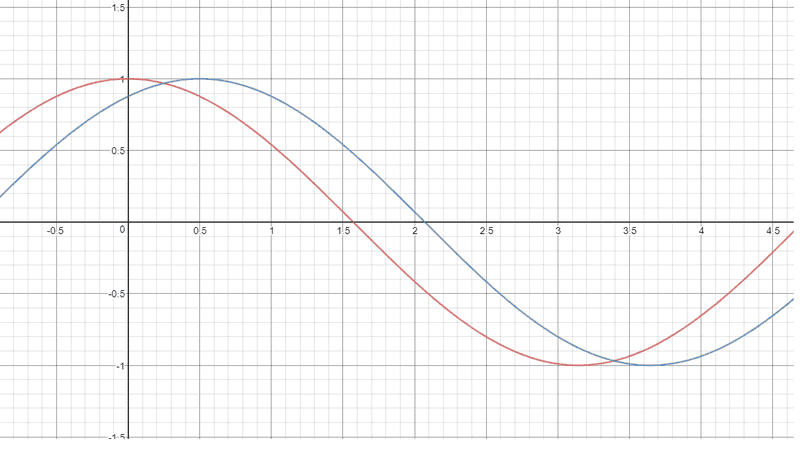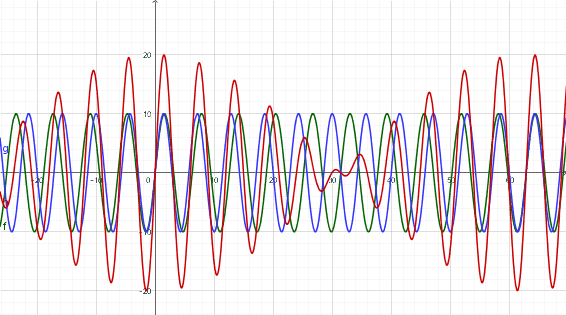# What is meant by natural frequency?

Applying it at any other frequency will sometimes oppose the motion. As the two frequencies drift in phase with each other, they will sometimes be in phase, reinforcing the motion, and other times out of phase, opposing the motion.
Like this graph right? At x=0.25 they are both opposing in motion, then they both descend together, and then oppose againFactChecker
Gold Member
No. At x=0.25, they are both positive and add together. They oppose in 1.6 < x < 2.0, where one is positive and the other is negative. In general, they add together instead of opposing. They could better synchronized, but not by much.
In the diagram below, the blue and green lines are two different frequencies and the red line is their sum. The red line shows that the blue and green reinforce each other at x=0 and phase drift so that they almost cancel each other at x=30.jbriggs444
Homework Helper
So to summarize, to achieve resonance, all you have to do is apply the force at times where it doesn't oppose motion. Applying it at any other frequency will oppose motion. Is this right?
If you apply the force at exactly the right frequency but at exactly the wrong phase, then you will be opposing motion despite having the right frequency. Over time the motion will gradually stop and then re-start, now in-phase with your force. If the oscillator is not damped, this motion will increase without bound.

If you have a damped oscillator and are pushing at either the wrong phase or not quite the right frequency then the same basic effect applies. The oscillator will gradually shift to a pattern that matches your driving rhythm. The magnitude of the resulting oscillations will depend on how much damping there is and on how closely the frequencies match.

•